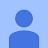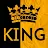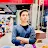APP Information

 Download Version 2.0.1 (3) Apk Size 2.64 MB App Developer Edu Technologies Malware Check TRUSTED Install on Android 4.1.x and up App Package com.edutechnologies.physicsformulas.apk MD5 72980a68d7c7609feac2ee002cd08e2c Rate 5# Get Familiar with Physics Formulas with this Android Application

Becoming successful in Physics requires extensive knowledge of theoretical formulas and a solid understanding of their different applications. For the students aspiring to conquer their physics course or even those who are already in the line of work, having Physics Formulas easily accessible and organized is a must. Fortunately, understanding the principles of physics is now available in a mobile format. With the Physics Formulas Android application, you have access to instructions, formulas and equations right in the palm of your hand.

Intended for both high school and university students studying Physics, this application simplifies the difficult concepts that often appear in textbooks. From the basics of kinematics and forces to more advanced topics like energy, momentum and electricity, this app will quickly become your biggest supporter when it comes to mastering Physics. It contains over 100 of the most important formulas and equations, neatly organized into six different sections.

The app also provides detailed graphical representations and diagrams, making it easier and more enjoyable to learn. Whether you are a physicist or just need some assistance in Physics class, this application will definitely help you stay organized and accelerate your progress. Even for experienced professionals, the app can serve as a refresher or as a tool for conducting further research. Having the fundamentals neatly organized and accessible is always a great option.

Getting this application on your Android device is free and quite easy. Physics Formulas is available for devices running Android 2.2 or later and it won't take more than a few minutes to install. Start mastering the principles of Physics and you will soon be the most knowledgeable individual around.

### App Description

```Physics Formulas

The app lists all the important Physics formulas. Its very useful for student to save valuable time.

This App contains following formulas :
Doppler Effect Formula
Force Formula
Work
Total Work Done
Power with Work
Power with Displacement
Power with Velocity
Kinetic Energy
Potential Energy
Centripetal (Centrifugal) Acceleration
Concave Mirror Magnification
Centripetal (Centrifugal) Force
Circular Velocity
Velocity
Average Velocity
Displacement or Distance
Kinetic Friction
Static Friction
Newton's Law of Gravitation
Kepler's Third Law
Acceleration due to Gravity
Escape Velocity
Hooke's Law
Elastic Potential Energy
Newton's Second Law of Motion
Impulse with Velocity
Impulse with Time
Momentum with Velocity
Momentum with Time
Moment Force, Lever Arm Length
Simple Pendulum
Physical Pendulum
Torque
Density
Einstein Mass Energy
Stress
Strain
Youngs Modulus
Ups Power Requirement
Doppler Effect
Wood Density
Differential Pressure Measurement
Sound Pressure Level
Sound Intensity Level
Sound Power Emitted
Sound Wavelength
Free Fall
Noise Pollution Level
Battery Storage Rating
Celsius to Fahrenheit Conversion
Fahrenheit to Celsius Conversion
True Porosity
Souders Brown Equation
Concave Mirror Equation
Constant Q Transform
Horsepower (HP)
Moment of Inertia
Transverse Strength of a Material
Standard Surface Factor
Rectangular Tank Capacity
Torsional Pendulum
Apparent Porosity
Kinematic Viscosity
Speed of Sound
Cylindrical Tank Capacity
Lensmaker's Equation
Podmore Factor
Leaf Spring Rate
Critical Frequency
Brinell Hardness Number (BHN)
Voltage Divider Rule
Electrodialysis (ED)
Amount of Substance
Angular Acceleration
Battery Charging Time
Kva (Kilovolt-amps)
Newton's Law of Cooling
Infusion with Calibrated Tubing
RMS Noise Voltage
Milling Speed, Feed Rate
Mass Flow Rate
Drift Velocity
Gsm of Paper
Helical Spring Rate
D Exponent
Gravitational Potential Energy
Damped Harmonic Oscillation
Forced Harmonic Oscillation
Quantum Harmonic Oscillator
Acoustic Suspension Enclosure
Sound Decibel Distance
Offset Frequency Speed Error
Motor Top Speed RPM
Power
Speedometer Calibration
Vehicles Angle, Angular Rate
One Dimensional Motion with Constant Acceleration
Kirchhoff's Voltage Law (KVL) / Loop Rule
Wien's Displacement Law
HP (Horsepower) to Amps Conversion
Graham's Law of Effusion
Snell's Law Refraction
Vehicle Speed Error
Root Mean Square Velocity Calculator
One Dimensional Motion Velocity
Acceleration Due to Gravity on Outside Earth
Boltzmann Gas Constant
De Broglie Wavelength
Average Translational Kinetic Energy
Energy of Photon
Nature of Light
Tidal Force
Angular Linear Velocity
Planck's Constant
Vector Displacement
Average Acceleration
Kinematic Displacement By Velocity and Time
Focal Length of Optical Convex
Magnitude of Vector
Moment of Inertia of a Point Mass for Rod
Work for Linear Motion
Sound Wave Velocity
Calculate Time Duration from Earth to Moon
Ceramic Transverse Strength
Inelastic Collision
Elastic Collision
Maximum Height of Projectile
Solid Shaft Maximum Torque
Solid Shaft Diameter
Shear Stress in Shaft
Lowest Spring Resonant Frequency
Hollow Shaft Maximum Torque
Total Energy
Work Done by Frictional Force
Tangential Velocity
Peukerts Law
Stokes Law
Newton's Third Law of Motion
Horizontal and Vertical Component
Convex Mirror Equation
Apparent Temperature
Inverse Square Law
Hull Speed
Vickers Hardness
Soil Moisture / Water Content
Muzzle Velocity
Inverse Square Law
Lever Effort Force
Aggregates Volume
Ampere
Pulse Repetition Frequency

This is an app designed for Students for quick access to Physics Formulas. Physics formulas can be memorize. So here is a simple way to refer to them and sharpen your Physics skills.
```

### More Android Apps to Consider

1.easy to understanding

2.Flimaker

3.Sarika Bajaj

4.Gouri Shankar

amazing

5.Daniel Hamammi

👍 good

6.Zeshan Ahmd

improve

7.8.9.10.